PV versus T graph of equal masses of ${\mathrm{H}}_{2}$$\mathrm{He}$ and ${\mathrm{O}}_{2}$ is shown in fig. Choose the correct alternative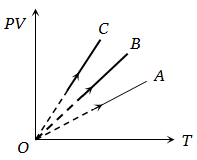1. C corresponds to ${\mathrm{H}}_{2}$, B to $\mathrm{He}$ and A to ${\mathrm{O}}_{2}$

2. A corresponds to $\mathrm{He}$, B to ${\mathrm{H}}_{2}$ and C to ${\mathrm{O}}_{2}$

3. A corresponds to $\mathrm{He}$, B to ${\mathrm{O}}_{2}$ and C to ${\mathrm{H}}_{2}$

4. A corresponds to ${\mathrm{O}}_{2}$, B to ${\mathrm{H}}_{2}$ and C to ${\mathrm{H}}_{2}$

High Yielding Test Series + Question Bank - NEET 2020

Difficulty Level:

Pressure versus temperature graph of an ideal gas at constant volume V of an ideal gas is shown by the straight line A. Now mass of the gas is doubled and the volume is halved, then the corresponding pressure versus temperature graph will be shown by the line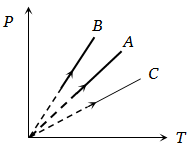1. A

2. B

3. C

4. None of these

High Yielding Test Series + Question Bank - NEET 2020

Difficulty Level:

Two different isotherms representing the relationship between pressure P and volume V at a given temperature of the same ideal gas are shown for masses ${\mathrm{m}}_{1}$ and ${\mathrm{m}}_{2}$ of the gas respectively in the figure given, then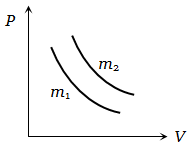1.

2.

3.

4. None of these

High Yielding Test Series + Question Bank - NEET 2020

Difficulty Level:

Two different masses m and 3m of an ideal gas are heated separately in a vessel of constant volume, the pressure P and absolute temperature T, graphs for these two cases are shown in the figure as A and B. The ratio of slopes of curves B to A is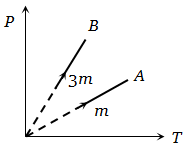1.  3 : 1

2.  1 : 3

3.  9 : 1

4.  1 : 9

High Yielding Test Series + Question Bank - NEET 2020

Difficulty Level:

Under constant temperature, graph between $\mathrm{P}$ and $1/\mathrm{V}$ is

1. Parabola

2. Hyperbola

3. Straight line

4. Circle

High Yielding Test Series + Question Bank - NEET 2020

Difficulty Level:

Which one the following graphs represents the behaviour of an ideal gas

1.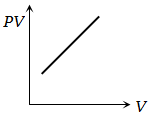2.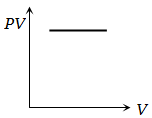3.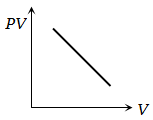4.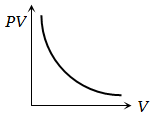High Yielding Test Series + Question Bank - NEET 2020

Difficulty Level:

The curve between absolute temperature and ${{\mathrm{v}}^{2}}_{\mathrm{rms}}$ is

1.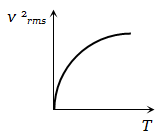2.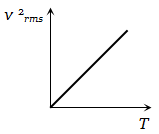3.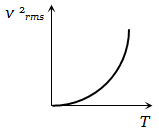4.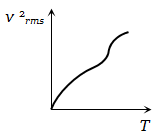High Yielding Test Series + Question Bank - NEET 2020

Difficulty Level:

Volume-temperature graph at atmospheric pressure for a monoatomic gas  is

1.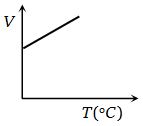2.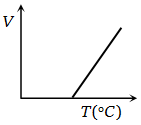3.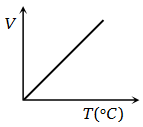4.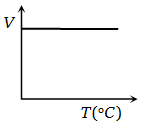High Yielding Test Series + Question Bank - NEET 2020

Difficulty Level:

Assertion: On reducing the volume of a gas at constant temperature, the pressure of the gas increases.

Reason: At constant temperature according to Boyle’s law, volume is inversely proportional to pressure

1. If both the assertion and the reason are true and the reason is a correct explanation of the assertion
2. If both the assertion and reason are true but the reason is not a correct explanation of the assertion
3. If the assertion is true but the reason is false
4. If both the assertion and reason are false

Concept Questions :-

Ideal gas

Difficulty Level:

Assertion: Number of air molecules in a room in winter is more than the number of molecules in the same room in summer.

Reason: At a given pressure and volume, the number of molecules of a given mass of a gas is directly proportional to the absolute temperature.

1. If both the assertion and the reason are true and the reason is a correct explanation of the assertion
2. If both the assertion and reason are true but the reason is not a correct explanation of the assertion
3. If the assertion is true but the reason is false
4. If both the assertion and reason are false

Concept Questions :-

Kinetic energy of gas